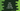# Different ways to iterate through a Dart map# Dart programs to iterate through a map :

Dart map is used to hold key-value pairs. We can have any type for the key or value. The key is unique. We can’t have two equal keys for a dart map. But we can have multiple same values.

You can iterate through the key-value pairs, or only the keys or only the values. Mainly forEach and for-in loop are used for it. In this post, I am listing down a couple of different ways to achieve it. Go through the code and if you have any other method in mind, leave a comment below.

### 1. forEach to iterate key-value pairs of a dart map :

forEach takes one function as its parameter in dart. You can use two parameters in the function to get both key and value of a map :

``````main() {
var numMap = Map();
numMap["one"] = 1;
numMap["two"] = 2;
numMap["three"] = 3;
numMap["four"] = 4;
numMap["five"] = 5;

numMap.forEach((k, v) => print("Key : \$k, Value : \$v"));
}``````

It will print all keys and values of numMap :

``````Key : one, Value : 1
Key : two, Value : 2
Key : three, Value : 3
Key : four, Value : 4
Key : five, Value : 5``````

### for-in to iterate keys and values :

You can get the value of a dart map if you know the key. Using one for-in loop, we can iterate through the keys and for each key, we can get the value :

``````main() {
var numMap = Map();
numMap["one"] = 1;
numMap["two"] = 2;
numMap["three"] = 3;
numMap["four"] = 4;
numMap["five"] = 5;

for (var k in numMap.keys) {
print("Key : \$k, value : \${numMap[k]}");
}
}``````

The output is similar to the above example.

### 3. forEach to print key-values using keys :

Using forEach, we can iterate only through the keys and for each key, we can print the value :

``````main() {
var numMap = Map();
numMap["one"] = 1;
numMap["two"] = 2;
numMap["three"] = 3;
numMap["four"] = 4;
numMap["five"] = 5;

numMap.keys.forEach((k) => print("Key : \$k, Value : \${numMap[k]}"));
}``````

If you want both key and value, you can go with the first method instead of this.

### 4. Iterate through the values :

.values returns one iterator for map values. You can use one forEach or for-in to iterate through these values. Example using for in :

``````main() {
var numMap = Map();
numMap["one"] = 1;
numMap["two"] = 2;
numMap["three"] = 3;
numMap["four"] = 4;
numMap["five"] = 5;

for (var v in numMap.values) {
print(v);
}
}``````

If you don’t need the keys, you can use this approach to iterate only through the values.

### 5. Using entries :

Entry is a key-value pair that represents one entry in a map. We can get the key and value using entry.key and entry.value. .entries returns one iterable of the map entries. We can use one for in method or forEach method to iterate through these entries and get the key-values :

``````main() {
var numMap = Map();
numMap["one"] = 1;
numMap["two"] = 2;
numMap["three"] = 3;
numMap["four"] = 4;
numMap["five"] = 5;

for (MapEntry e in numMap.entries) {
print("Key \${e.key}, Value \${e.value}");
}
}``````

Using forEach :

``````main() {
var numMap = Map();
numMap["one"] = 1;
numMap["two"] = 2;
numMap["three"] = 3;
numMap["four"] = 4;
numMap["five"] = 5;

numMap.entries.forEach((e) => print("Key \${e.key}, Value \${e.value}"));
}``````